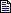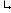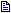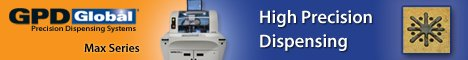Printed Circuit Board Assembly & PCB Design Forum

SMT electronics assembly manufacturing forum.

# Resistance value level of lead-free solder jointWhat's the resistance value level of lead-free joint?Could a... - May 23, 2003Resistance: Tables of wire gauge often provide a resistance ... - May 26, 2003Thanks Dave. It's very nice of you. Jed ... - May 27, 2003Dave- Thanks for the NIST Site.....Again, another useful nu... - May 29, 2003

jedduan

Resistance value level of lead-free solder joint | 23 May, 2003

What's the resistance value level of lead-free joint?Could anyone share me with the information? Thanks in advance Jed

Resistance value level of lead-free solder joint | 26 May, 2003

Resistance: Tables of wire gauge often provide a resistance measure of some kind for each gauge. That way we can estimate the resistance of any length of wire we might be interested in. Calculating trace resistance is only slightly more complicated. Metals have a characteristic called �resistivity� (r) sometimes also called �specific resistance.� Resistivity is defined by the relationship R = r * l / a where R = resistance in Ohms, l = length and a = cross-sectional area. Units for resistivity are Ohm-unit where �unit� is the unit system for l and a. The resistivity for pure copper is often given as: r = 1.724 microhm-cm, or r = .6788 microhm-in Therefore, the resistance of any copper trace can be found in a fairly straightforward manner by taking this value for resistivity, dividing it by the cross-sectional area of the trace, and then multiplying by the length of the trace. There is a minor complication here, however. Resistivity changes with temperature! Therefore, resistivity constants are usually given for a specific temperature, and that usually is 20*C. So this calculation will give the resistance of the trace at 20*C. [UltraCAD]

Tables of resistivity of solders are available on the net. For instance look at table 2.2.19, here: http://www.boulder.nist.gov/div853/lead%20free/props01.html

For a test method look here: http://www.ipc.org/4.0_Knowledge/4.1_Standards/test/2.5.17e.pdf

jedduan

Resistance value level of lead-free solder joint | 27 May, 2003

Thanks Dave. It's very nice of you.

Jed

Resistance value level of lead-free solder joint | 29 May, 2003

Dave- Thanks for the NIST Site.....Again, another useful nugget!!!

Cal